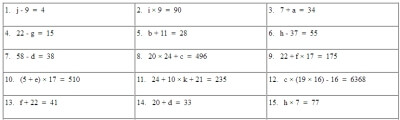Worksheets and No Prep Teaching Resources
Math Worksheets
Algebra Worksheets

# Equation WorksheetsEquations: Algebra Basics
Translating Verbal Statements into Equations
Solving Simple Equations Using Inverse Operations
Solving Multiplication and Division Equations
Solving Equations with 2 to 4 Numbers
More 2 to 4 Number Equations
Write a Word Problem for Each Equation
Equations Final Review!

Equations: Algebra Basics Crossword Puzzles
Translating Verbal Statements into Equations Crossword Puzzle
Solving Simple Equations Using Inverse Operations Crossword Puzzle
Solving Addition and Subtraction Equations Crossword Puzzle
Solving Multiplication and Division Equations Crossword Puzzle
Solving Equations with 2 to 4 Numbers Crossword Puzzle
More 2 to 4 Number Equations Crossword Puzzle
Equations Final Review Crossword Puzzle!